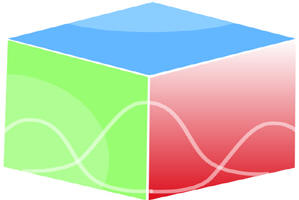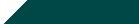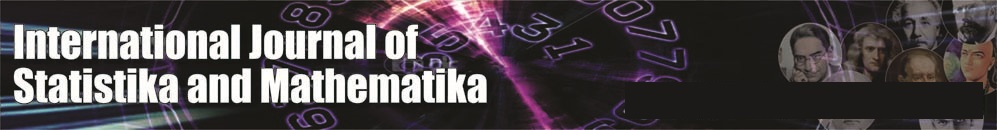``Home| Journals | Statistics Online Expert | About Us | Contact UsUntitled Document

[

Profit- function of two-unit dissimilar warm standby system with Erlang-K failure time distribution subject to fluctuations in temperatures

Ashok Kumar Saini*

*Associate Professor, Department of Mathematics, B.L.J.S. College, Tosham, Bhiwani, Haryana, INDIA.

Email:

Research Article

Abstract               Introduction: In Reliability engineering literature most of the authors have taken failure time distribution as exponential. In the present paper we have taken two-dissimilar unit warm cold standby system with failure time distribution as Erlang-K with general repair time distributions. The Role of temperature under which the system operates plays significant role on its working. We are considering system under (i) mild temperature and (ii) steep high temperature causing different phase type Erlang-K failure time distribution requiring different types of repair facilities. Using semi Markov regenerative point technique we have calculated different reliability characteristics such as MTSF, reliability of the system, availability analysis in steady state, busy period analysis of the system under repair, expected number of visits by the repairman in the long run and profit-function. Special case by taking failure and repair as exponential have been derived and graphs are drawn.

Keyword: warm standby, Erlang-K distribution, mild temperature, steep high temperature.

INTRODUCTION

Assumptions

• The failure time distribution is Erlang-K whereas the repair time distribution is arbitrary of two non-identical units.
• The repair facility is of four types :

Type I, II repair facility

• when failure of phase type 1 and phase Type 2 due to mild and steep high temperature of one unit with rates , respectively and

Type III, IV repair facility

• when failure of phase Type 3, phase type 4 due to mild and steep high temperature of the second unit respectively.
• The repair starts immediately upon failure of units and the repair discipline is FCFS.
• The repairs are perfect and starts immediately as soon as the temperature of the system becomes normal. The temperature of both the units do not go steep high.
• The failure of a unit is detected immediately and perfectly.
• The switches are perfect and instantaneous.
• All random variables are mutually independent.

SYMBOLS FOR STATES OF THE SYSTEM

Superscripts: O, WS, SO, F, SFO

Operative, Warm Standby, Stops the operation, Failed, Switch failed but operable respectively

Subscripts: nt, ut,ur, wr, uR

No steep high temp., under steep high temp., under repair, waiting for repair, under repair continued respectively

Up states: 0, 1, 2, 9; down states – 3,4,5,6,7,8,10,11

Regeneration point: 0,1,2,4,7,10

States of the System

0(Ont , WSnt)

One unit is operative and the other unit is warm standby and there are no steep high temp. in both the units.

1(SOnt , Ont)

The operation of the first unit stops automatically due to steep high temp. and warm standby units starts operating.

2(Fur , Ont)

The first one unit fails and undergoes repair after the steep high temp. are over and the other unit continues to be operative with no steep high temp..

3(FuR , SOut)

The repair of the first unit is continued from state 2 and in the other unit steep high temp. occur and stops automatically due to steep high temp.

4(Fur, SOut)

The one unit fails and undergoes repair after the steep high temp. are over and the other unit also stops automatically due to steep high temp..

5(FuR, Fwr) The repair of the first unit is continued from state 4 and the other unit is failed due to steep high temp.in it and is waiting for repair.

Figure 1: The State Transition Diagram

7(SOnt , SFOnt,ur)

The operation of the first unit stops automatically due to steep high temp. and during switchover to the second unit switch fails and undergoes repair.

8(Fwr, SFOnt,ur)

The repair of failed switch is continued from state 7 and the first unit is failed after steep high temp. and waiting for repair.

9(Ont, SOut)

The first unit is operative and the warm standby dissimilar unit is under steep high temp.

10(SOnt, Fur)

The operation of the first unit stops automatically due to steep high temp. and the second unit switch fails and undergoes repair after the steep high temp. is over.

11(Fwr, FuR)

The repair of the second unit is continued from state 10 and the first unit is failed after and waiting for repair.

TRANSITION PROBABILITIES

Simple probabilistic considerations yield the following expressions:

p01 =  , P07 =

p09 =  , p12 =  , p14 =

P23= G1*( λ1) , P22(3) = G1*( λ1) , P72 = G2*( λ3) , P728 = G2*( λ3)= P78

Also other values can be defined.

We can easily verify that

p01 + p02 = 1, p13 + p14 = 1, p23 + p23(1) + p24= 1 , p30 + p336 = 1,

p43+ P435 = 1                                                                                                                                                                                        (1)

And mean sojourn time are

µ0 = E(T) =                                                                                                                                                              (2)

Mean Time To System Failure

We can regard the failed state as absorbing

,

(3-5)

Taking Laplace-Stiltjes transform of eq. (3-5) and solving for

= N1(s) / D1(s)                                                                                                                                                                  (6)

Where

N1(s) = {  +

D1(s) = 1 -

Making use of relations (1) and (2) it can be shown that  =1 , which implies

that  is a proper distribution.

MTSF = E [T] =     = (D1(0) - N1(0)) / D1 (0)

s=0

= (+p01  + p01 p12  + p09 ) / (1 - p01 p12 p20 )

where

+  +  ,  + , + (3) ,

AVAILABILITY ANALYSIS

Let Mi(t) be the probability of the system having started from state I is up at time t without making any other regenerative state belonging to E. By probabilistic arguments, we have The value of M0 (t), M1(t), M2(t), M4(t) can be found easily.

The point wise availability Ai(t) have the following recursive relations

A0(t) = M0(t) + q01(t)[c]A1(t) + q07(t)[c]A7(t) + q09(t)[c]A9(t)

A1(t) = M1(t) + q12(t)[c]A2(t) + q14(t)[c]A4(t) , A2(t) = M2(t) + q20(t)[c]A0(t) + q22(3)(t)[c]A2(t)

A4(t) = q46(3)(t)[c]A6(t) , A6(t) = q60(t)[c]A0(t)

A7(t) = (q72(t)+ q72(8)(t)) [c]A2(t) + q74 (t)[c]A4(t)

A9(t) = M9(t) + q9,10(t)[c]A10(t) , A10(t) = q10,2(t)[c]A2(t) + q10,2(11)(t)[c]A2(t)                                                                             (7-14)

Taking Laplace Transform of eq. (7-14) and solving for

= N2(s) / D2(s)                                                                                                                                                                 (15)

Where

N2(s) = (1 -  22(3)(s)) {  0(s) + 01(s) 1(s) + 09(s) 9(s)}+ 2(s){ 01(s) 42(s) + 07(s)72(s) +  73(8)(s)) +  09 (s) 9,10 (s)( 10,2 (s) + 10,2(11)(s))}

D2(s) = (1 -  22(3)(s)) { 1 -  46(5)(s) 60(s) ( 01(s) 44 (s) +  07(s) 74(s))

- 20(s)01(s)12(s)07(s)(  72(s)) +  72(8)(s) +  09 (s) 9,10 (s)

( 10,2 (s) + 10,2(11)(s))}

A0 =  =  =

Using L’ Hospitals rule, we get

A0 =  =                                                                                                                                           (16)

Where

N2(0)= p20(0(0) + p011(0) + p09 9(0) ) + 2(0) (p01p12 + p07 (p72

+ p728 + p09 ))

D2(0) = p20{  + p01 + (p01 p14 + p07 p74 )+ p07  + p07  + p09()

+ { 1- ((p01p14 + p07 p74 )}

,  ,

The expected up time of the system in (0,t] is

(t) =  So that                                                                                                       (17)

The expected down time of the system in (0,t] is

(t) = t- (t) So that                                                                                                                   (18)

The expected busy period of the server for repairing the failed unit under steep high temperature in (0,t]

R0(t) = S0(t) + q01(t)[c]R1(t) + q07(t)[c]R7(t) + q09(t)[c]R9(t)

R1(t) = S1(t) + q12(t)[c]R2(t) + q14(t)[c]R4(t) ,

R2(t) = q20(t)[c]R0(t) + q22(3)(t)[c]R2(t)

R4(t) = q46(3)(t)[c]R6(t), R6(t) = q60(t)[c]R0(t)

R7(t) = (q72(t)+ q72(8)(t)) [c]R2(t) + q74 (t)[c]R4(t)

R9(t) = S9(t) + q9,10(t)[c]R10(t), R10(t) = q10,2(t) + q10,2(11)(t)[c]R2(t) (19-26)

Taking Laplace Transform of eq. (19-26) and solving for

= N3(s) / D2(s)                                                                                                                                                                  (27)

Where

N2(s) = (1 -  22(3)(s)) {  0(s) + 01(s) 1(s) + 09(s) 9(s)} and D2(s) is already defined.

In the long run, R0 =  (28)

where N3(0)= p20(0(0) + p011(0) + p09 9(0) ) and D2(0) is already defined.

The expected period of the system under steep high temperature in (0,t] is

(t) =  So that

The expected Busy period of the server for repair of dissimilar units by the repairman in (0,t]

B0(t) = q01(t)[c]B1(t) + q07(t)[c]B7(t) + q09(t)[c]B9(t)

B1(t) = q12(t)[c]B2(t) + q14(t)[c]B4(t) , B2(t) = q20(t)[c] B0(t) + q22(3)(t)[c]B2(t)

B4(t) = T4 (t)+ q46(3)(t)[c]B6(t) , B6(t) = T6 (t)+ q60(t)[c]B0(t)

B7(t) = (q72(t)+ q72(8)(t)) [c]B2(t) + q74 (t)[c]B4(t)

B9(t) = q9,10(t)[c]B10(t) , B10(t) = T10 (t)+ (q10,2(t) + q10,2(11)(t)[c]B2(t)                                                                                             (29- 36)

Taking Laplace Transform of eq. (29-36) and solving for

= N4(s) / D2(s)                                                                                                                                                                  (37)

Where

N4(s) = (1 -  22(3)(s)) { 01(s)14(s) 4(s) + 46 (5)(s) 6(s)) + 07(3)(s) 74(s)( 4(s)

+   46(5)(s) 6(s))+ 09(s)09,10(s)  10(s) )

In steady state, B0 =                                                                                                                                                       (38)

where N4(0)= p20 {( p01 p14 + p07 p74) (4(0) +6(0)) + p09 10(0) } and D2(0) is already defined.

The expected busy period of the server for repair in (0,t] is

(t) =  So that                                                                                                                      (39)

The expected Busy period of the server for repair of switch in (o, t]

P0(t) = q01(t)[c]P1(t) + q07(t)[c]P7(t) + q09(t)[c]P9(t)

P1(t) = q12(t)[c]P2(t) + q14(t)[c]P4(t) , P2(t) = q20(t)[c]P0(t) + q22(3)(t)[c]P2(t)

P4(t) = q46(3)(t)[c]P6(t) , P6(t) = q60(t)[c]P0(t)

P7(t) = L7(t)+ (q72(t)+ q72(8)(t)) [c]P2(t) + q74 (t)[c]P4(t)

P9(t) = q9,10(t)[c]P10(t) , P10(t) = (q10,2(t) + q10,2(11)(t))[c]P2(t)                                                                                          (40-47)

Taking Laplace Transform of eq. (40-47) and solving for

= N5(s) / D2(s)                                                                                                                                                                  (48)

where N2(s) = 07(s )  7(s) 1 -  22(3)(s)) and D2(s) is defined earlier.

In the long run, P0 =                                                                                                                                                        (49)

where N5(0)= p20 p07 4(0) and D2(0) is already defined.

The expected busy period of the server for repair of the switch in (0,t] is

(t) =  So that                                                                                                                        (50)

The expected number of visits by the repairman for repairing the different units in (0,t]

H0(t) = Q01(t)[c]H1(t) + Q07(t)[c]H7(t) + Q09(t)[c]H9(t)

H1(t) = Q12(t)[c][1+H2(t)] + Q14(t)[c][1+H4(t)] , H2(t) = Q20(t)[c]H0(t) + Q22(3)(t)[c]H2(t)

H4(t) = Q46(3)(t)[c]H6(t) , H6(t) = Q60(t)[c]H0(t)

H7(t) = (Q72(t)+ Q72(8)(t)) [c]H2(t) + Q74 (t)[c]H4(t)

H9(t) = Q9,10(t)[c][1+H10(t)] , H10(t) = (Q10,2(t)[c] + Q10,2(11)(t))[c]H2(t) (51-58)

Taking Laplace Transform of eq. (51-58) and solving for

= N6(s) / D3(s)                                                                                                                                                                   (59)

Where

N6(s) = (1 –  22(3)*(s)) { 01(s)12(s)14(s))  09 (s) 9,10 (s)}

D3(s) = (1 -  22(3)*(s)) { 1 - (01(s) 14 (s) +  07(s) 74(s))46(5)*(s) 60(s)}

- 20(s)01(s)12(s)07(s)( 72(s)) +  72(8)(s) +

09 (s)9,10 (s) ( 10,2 (s) +Q 10,2(11)*(s))}

In the long run, H0 =                                                                                                                                                       (60)

where N6(0)= p20 (p01 + p09) and D’3(0) is already defined.

The expected number of visits by the repairman for repairing the switch in (0,t]

V0(t) = Q01(t)[c]V1(t) + Q07(t)[c]V7(t) + Q09(t)[c]V9(t)

V1(t) = Q12(t)[c]V2(t) + Q14(t)[c]V4(t) , V2(t) = Q20(t)[c]V0(t) + Q22(3)(t)[c]V2(t)

V4(t) = Q463(t)[c]V6(t) , V6(t) = Q60(t)[c]V0(t)

V7(t) = (Q72(t)[1+V2(t)]+ Q728(t)) [c]V2(t) + Q74 (t)[c]V4(t)

V9(t) = Q9,10(t)[c]V10(t) , V10(t) = (Q10,2(t) + Q10,211(t))[c]V2(t)                                                                                        (61-68)

Taking Laplace-Stieltjes transform of eq. (61-68) and solving for

= N7(s) / D4(s)                                                                                                                                                                  (69)

where N7(s) =  07 (s) 72 (s) (1 –  22(3)*(s)) and D4(s) is the same as D3(s)

In the long run, V0 =                                                                                                                                                       (70)

where N7(0)= p20 p07 p72 and D’3(0) is already defined.

COST BENEFIT ANALYSIS

The cost-benefit function of the system considering mean up-time, expected busy period of the system under steep high temp. when the units stops automatically, expected busy period of the server for repair of unit and switch, expected number of visits by the repairman for unit failure, expected number of visits by the repairman for switch failure.

The expected total cost-benefit incurred in (0,t] is

C (t) = Expected total revenue in (0,t] expected total repair cost for switch in (0,t]

• expected total repair cost for repairing the units in (0,t ]
• expected busy period of the system under steep high temperature when the units automatically stop in (0,t]
• expected number of visits by the repairman for repairing the switch in (0,t]
• expected number of visits by the repairman for repairing of the units in (0,t]

The expected total cost per unit time in steady state is

C = =

= K1A0 - K2P0 - K3B0 - K4R0 - K5V0 - K6H0

Where

K1: revenue per unit up-time,

K2: cost per unit time for which the system is under switch repair

K3: cost per unit time for which the system is under unit repair

K4: when units automatically stop. cost per unit time for which the system is under steep high temp.

K5: cost per visit by the repairman for which switch repair,

K6: cost per visit by the repairman for units repair.

CONCLUSION

After studying the system , we have analysed graphically that when the failure rate, steep high temperature rate increases, the MTSF and steady state availability decreases and the cost function decreased as the failure increases.

REFERENCES

1. Barlow, R.E. and Proschan, F., Mathematical theory of Reliability, 1965; John Wiley, New York.
2. Dhillon, B.S. and Natesen, J, Stochastic Anaysis of outdoor Power Systems in fluctuating environment, Microelectron. Reliab. .1983; 23, 867-881.
3. Gnedanke, B.V., Belyayar, Yu. K. and Soloyer , A.D. , Mathematical Methods of Relability Theory, 1969 ; Academic Press, New York.
4. Goel, L.R., Sharma,G.C. and Gupta, Rakesh Cost Analysis of a Two-Unit standby system with different weather conditions, Microelectron. Reliab. , 985; 25, 665-659.
5. Goel, L.R. ,Sharma G.C. and Gupta Parveen , Stochastic Behaviour and Profit Anaysis of a redundant system with slow switching device, Microelectron Reliab., 1986; 26, 215-219.